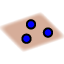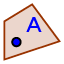# Geometry Notes 1.1: Points,Lines, and Planes

Undefined terms: A point is a location and is named by a capital letter. A line is made up of points and has no thickness or width. It is named with two letters representing points on the line, or a lowercase script letter. A plane is an infinite flat surface made up of points and is named by a capital script letter or by three letters representing non collinear points on a plane. Collinear points can be contained on a line. Coplanar points can be contained on a plane.
FIRST DIAGRAM: Drag the diagram around by moving the points (you can even make it spin!), and then wait for the class.
BREAK OUT ROOMS: remember to leave your microphone on, and talk through the problems with your partner. Compare answers, and discuss what you see. Do #1 - #4 on GeoGebra, and #1-#4 on your printed notes.

1. Name 3 collinear points

2. Name 3 noncollinear points:

3. Name a plane.

4. Name in a different way. (Choose all that apply)

Select all that apply
• A
• B
• C
• D
Now do #1-#4 on your printed notes together, and then: STOP! LEAVE YOUR BREAK-OUT ROOM AND GO BACK TO THE MAIN ROOM! (Don't do #5 yet.)
Next: With the teacher: Draw , () and then drag it. Notice how the part of the line that is behind the plane is dashed. Draw plane CEA (), and then drag it. Then delete the line and plane that you drew.
BREAK OUT ROOMS: remember to leave your microphone on, and talk through the problems with your partner. Compare answers, and discuss what you see. Do #5 - #12 on GeoGebra, and then do # 5 - #10 on your printed notes.

5. If possible, draw a line through E and C. Are E and C collinear?

Select all that apply
• A
• B

6. If possible, draw a line through E, G, and C. Are E, G, and C collinear?

Select all that apply
• A
• B

7. If possible, name two points that are non collinear, because you CANNOT draw a line through them.

8. If possible, draw a plane through D, B, and F. Are D, B, and F coplanar?

9. If possible, draw a plane through A, G, E, and B. Move the diagram around to see if the four points are on the plane. Are A, G, E, and B coplanar?

10. If possible, name 3 points that are NOT coplanar, because you CANNOT draw a plane through them.

SECOND DIAGRAM: Use points F, D, and E to drag the rectangular prism around. Then answer the following questions. Remember to discuss each problem with your partner-- microphones on, talk over the question.

11. Name the intersection of and .

12. Name the intersection of and (the lines are not shown)

Do #5 - #10 on your printed notes together, and then: Stop! Leave the breakout room and go to the main room.(Don't do #16 yet.)
Next we will practice drawing a 3 dimensional diagram in two dimensions, as though you are drawing on paper. How can you represent a plane? How can you represent a line or point that is NOT on that plane? First, practice with the teacher: a) Draw a plane that looks like the plane in the printed notes. ( using the polygon tool. () b) Draw two points on the plane, and draw the line through them. (and) c) Draw two points NOT on the plane, draw the line through them. (and) d) Use dashed segments to make it clear that that line is not on the plane. ()

## Try #16 on your own:

16. Use the Applet below. a) Draw two points, P and Q using thetool. b) Sketch using thetool. c) Add a point R on the line so that Q is between P and R.

## Try #17 on your own, except for d).

17. Use the Applet below. a) Draw a plane using thetool. b) Put point Q on the plane using thetool. c) Draw ﻿ so that it intersects the plane only once. d) We can use the segment tool to do a pretty good job of creating a dashed section.## Drawing a 3D diagram in two dimensions!

Now let's finish the PRINTED NOTES. Don't log off GeoGebra yet, though.